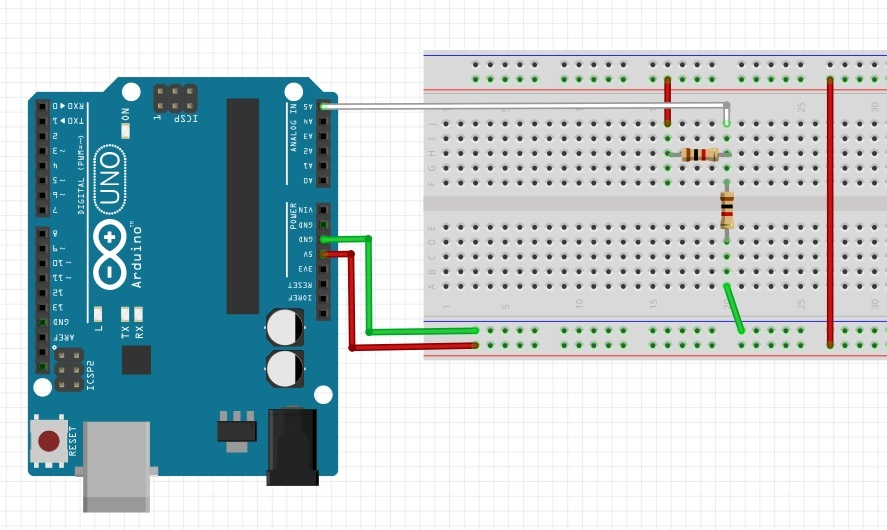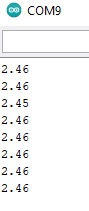This will show how to use analogRead to get a voltage from an circuit.  This circuit is a voltage divider.   I have two 1000 Ω resistors in series.  It will cut the voltage in half.  Without a multimeter it will show you have to display the analog (floating point) voltage.  A5 is the analog port that will read the value of the voltage divider.  EE: Resistor page shows you how resistors in circuits are calcuated.

analogWrite is 0 to 255 (8 bit).  analogRead is 0 to 1024 ( 10 bit). So to convert analogRead into a voltage.

(5. / 1023. ) *analogPort value.I will put the below code into the Arduino and copy the ino file up after building the circuit.